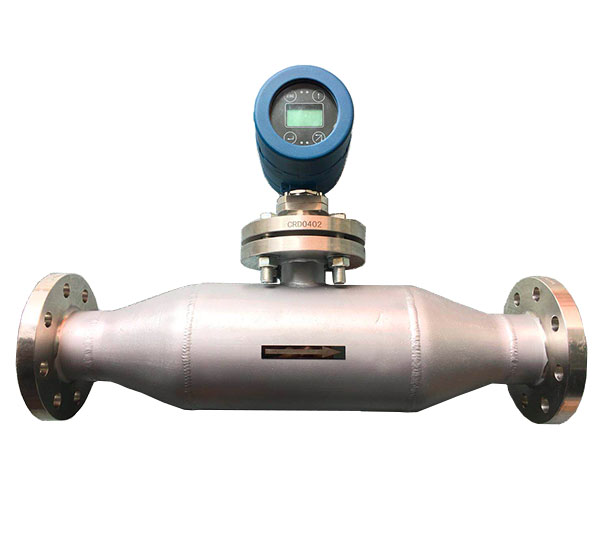D= K0+K1T+K2T2
D=未校准的被测介质密度(kg/m3)
T=振动频率(μs).

D= K0+K1T+K2T2
D=未校准的被测介质密度(kg/m3)
T=振动频率(μs).

K0、K1、K2=常量